Gravimetric exercises (2009) Elemental sulfur can be used to control outbreaks of powdery mildew on grapes. However, sulfur remaining on the grapes after harvest can be converted to a number of undesirable compounds during fermentation in wine production. A wine chemist uses atomic absorption spectroscopy to determine the amount of sulfur remaining on grapes. In a particular analysis, 100.0 g of grapes are treated with 100.0 mL of surfactant solution to remove the sulfur remaining on the grapes when they were harvested. 25.00 mL of this surfactant solution is treated to convert all of the sulfur to sulfate ions and then dried to produce an ash containing the sulfate ions. This ash is transferred to a 10.00 mL volumetric flask containing 2.00 mL of 200 mg/L solution of barium Ba2+ions. The volume of solution in the volumetric flask is then made up to the calibration line. A precipitate of BaSO4 forms and settles to the bottom of the volumetric flask. A small amount of the solution containing the unreacted Ba2+ ions is removed from the volumetric flask and analysed using atomic absorption spectroscopy. This solution gave an absorbance of 0.11. A calibration curve was prepared using standard solutions of 10, 20, 30 and 40 mg/L Ba2+ (aq).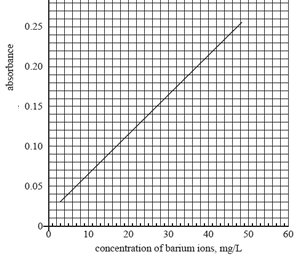a) Determine the concentration of barium ions remaining in the 10.00 mL sample solution. Hence determine the mass of barium ions, in mg, remaining in the 10.00 mL sample solution. Click here for solution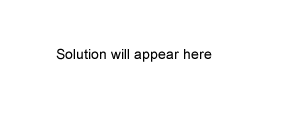b) Determine the amount of barium ions, in moles, that reacted to produce the barium sulfate precipitate. Click here for solution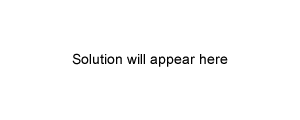c) Determine the mass of sulfur, in mg, remaining on the 100 g of harvested grapes.   Click here for solution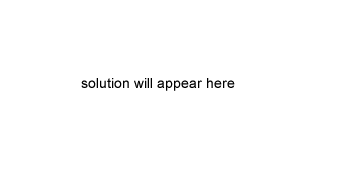d) The amount of sulfur remaining on the grapes can also be determined using gravimetric analysis. Give one reason why atomic absorption spectroscopy is a better way to determine the residual sulfur on the grapes compared to gravimetric analysis. Click here for solution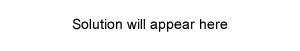Continue with another exercise from 2009   Continue with more gravimetric analysis exercises from past exam papers